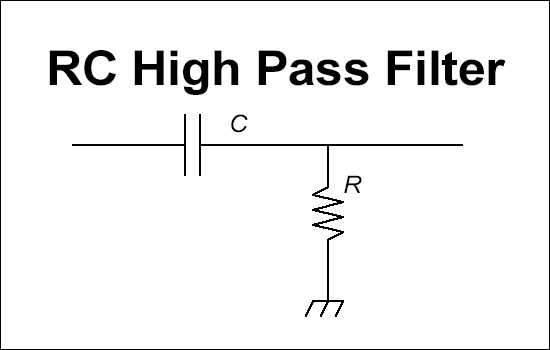# RC High Pass Filter - Frequency and Bode Plot Calculator

This tool calculates the crossover frequency for a RC high pass filter. In addition, it graphs the bode plot for magnitude in decibels and the phase in radians. The graph is unable to plot below 1Hz at the moment. The output frequency is rounded to the second decimal place. Please notify the admin if there are any bugs or requests.R:

C:

## Bode Plot

Bode plot for magnitude

Bode phase plot